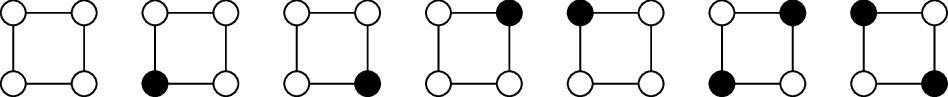# Blog

## The number of independent sets in an irregular graph

May 12, 2018

I am excited to announce our new paper, The number of independent sets in an irregular graph, coauthored with these three amazing collaborators:

Ashwin is a freshman at MIT, Mehtaab is a sophomore at MIT, and David is a junior at Harvard.

The paper solves a conjecture made by Jeff Kahn in 2001 concerning the number of independent sets in a graph.

An independent set of a graph is a subset of vertices with no two adjacent.Example: the complete list of independent sets of a cycle of length 4

Since many problems in combinatorics can be naturally phrased in terms of independent sets in a graph or hypergraph, getting good bounds on the number of independent sets is a problem of central importance.

Instead of giving the exact statement of the conjecture (now theorem), which you can find in the abstract of our paper, let me highlight a specific instance of a problem addressed by the theorem:

Question. Consider the family of graphs with no isolated vertices and

• exactly a third of the edges have degree 3 on both endpoints, and
• a third of the edges have degree 4 on both endpoints,
• the remaining third of the edges have degree 3 on one endpoint and degree 4 on the other endpoint.

What is the smallest constant c such that every n-vertex graph satisfying the above properties has at most $c^n$ independent sets?

In other words, letting $i(G)$ denote the number of independent sets and $v(G)$ the number of vertices of $G$, maximize the quantity $i(G)^{1/v(G)}$ over all graphs G in the above family.

(See the end of this post for the answer)

In summer 2009, as an undergraduate participating in the wonderful Research Experience for Undergraduates (REU) run by Joe Gallian in Duluth, MN, I learned about Kahn’s conjecture (somewhat accidentally actually, as I was working on a different problem that eventually needed some bounds on the number of independent sets, and so I looked up the literature and learned, slightly frustratingly at the time, that it was an open problem). It was on this problem that I had written one of my very first math research papers.

I had been thinking about this problem on and off since then. A couple years ago, Joe Gallian invited me to write an article for a special issue of the American Mathematical Monthly dedicated to undergraduate research (see my previous blog post on this article), where I described old and new developments on the problem and collected a long list of open problems and conjectures on the topic (one of them being Kahn’s conjecture).

This spring, I had the privilege with working with Ashwin, Mehtaab, and David, three energetic and fearless undergraduate students, finally turning Kahn’s conjecture into a theorem. Fearless indeed, as our proof ended up involving quite a formidable amount of computation (especially for such an innocent looking problem), and my three coauthors deserve credit for doing the heavy-lifting. I’ve been told that there had been many late night marathon sessions in an MIT Building 2 classroom where they tore apart one inequality after another.

This is certainly not the end of the story, as many more interesting problems on the subject remain unsolved—my favorite one being the analogous problem for colorings, e.g., instead of counting the number of independent sets, how about we count the number of ways to color the vertices of the graph with 3 colors so that no two adjacent vertices receive the same color? (See my previous blog post for some more discussion.)

Anyway, here is the the answer to the question above. The number of independent sets in an n-vertex graph satisfying the above properties is at most $c^n$, where the optimal constant for c is $15^{4/63} \times 23^{1/21} \times 31^{1/28} = 1.558\dots$, or, more helpfully, the maximum is attained by the following graph (in general, the theorem says that the maximizer is always a disjoint union of complete bipartite graphs):